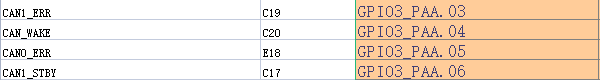# Config of can device tree

Hello, I encountered some problems when configuring the can device tree. I need your help. Thank you.
In the following settings, gpio_ can_ stb and gpio_ can_ en What are the functions of the two attributes?

``````mttcan@c310000 {
status = "okay";
gpio_can_stb = <&tegra_aon_gpio TEGRA_AON_GPIO(AA, 0) GPIO_ACTIVE_HIGH>;
gpio_can_en = <&tegra_aon_gpio TEGRA_AON_GPIO(AA, 1) GPIO_ACTIVE_HIGH>;
};

mttcan@c320000 {
status = "okay";
gpio_can_stb = <&tegra_aon_gpio TEGRA_AON_GPIO(AA, 6) GPIO_ACTIVE_HIGH>;
gpio_can_en = <&tegra_aon_gpio TEGRA_AON_GPIO(AA, 7) GPIO_ACTIVE_HIGH>;
};

``````

In my circuit, CAN0_ STBY(E17)、CAN0_ ERR(E18)、CAN1_ STBY(C17)、CAN1_ ERR (C19) are all connected to DGND. In this case, do I need to set the device tree above to：

``````mttcan@c310000 {
status = "okay";
gpio_can_stb = <&tegra_aon_gpio TEGRA_AON_GPIO(AA, 0) GPIO_ACTIVE_LOW>;
gpio_can_en = <&tegra_aon_gpio TEGRA_AON_GPIO(AA, 1) GPIO_ACTIVE_LOW>;
};

mttcan@c320000 {
status = "okay";
gpio_can_stb = <&tegra_aon_gpio TEGRA_AON_GPIO(AA, 6) GPIO_ACTIVE_LOW>;
gpio_can_en = <&tegra_aon_gpio TEGRA_AON_GPIO(AA, 7) GPIO_ACTIVE_LOW>;
};
``````

hello Yolomei,

it’s the device tree to configure default pin state, you may update those settings.
you may see-also Topic 63300 to enable and check CAN on TX2.
thanks

Still don’t understand. I found that no matter how I set these two attributes (high, low, or delete), they do not affect the use of the can bus.

``````mttcan@c310000 {
status = "okay";
gpio_can_stb = <&tegra_aon_gpio TEGRA_AON_GPIO(AA, 0) GPIO_ACTIVE_HIGH>;
gpio_can_en = <&tegra_aon_gpio TEGRA_AON_GPIO(AA, 1) GPIO_ACTIVE_HIGH>;
};

mttcan@c310000 {
status = "okay";
gpio_can_stb = <&tegra_aon_gpio TEGRA_AON_GPIO(AA, 0) GPIO_ACTIVE_LOW>;
gpio_can_en = <&tegra_aon_gpio TEGRA_AON_GPIO(AA, 1) GPIO_ACTIVE_LOW>;
};

mttcan@c310000 {
status = "okay";
//   gpio_can_stb = <&tegra_aon_gpio TEGRA_AON_GPIO(AA, 0) GPIO_ACTIVE_LOW>;
//   gpio_can_en = <&tegra_aon_gpio TEGRA_AON_GPIO(AA, 1) GPIO_ACTIVE_LOW>;
};
``````

hello Yolomei,

is the CAN interface up for the usage? may I know how you update the device tree?

After I finish setting in the device tree, use the following command to update the device tree:

``````sudo ./flash.sh -k kernel-dtb jetson-tx2 mmcblk0p1
``````

After updating the device tree, use the following command to test：

``````candump can0 &
cansend can0 123#abcdabcd
``````

hello Yolomei,

there’s cboot options for loading device tree via file system. it’s checking `/boot/extlinux/extlinux.conf` for the `FDT ` entry, if that’s specified, it’ll prior to `kernel-dtb` partition for loading device tree blob.

I don’t quite understand what you mean. What I want to ask is, gpio_ can_ stb and gpio_ can_ en What are the functions of the two attributes? Why do my settings of these two attributes not affect the test results of the can bus

hello Yolomei,

so, please ensure you’ve update device tree correctly.

Each time, it is retested after successful flash. So, where can I see the describe of these two attributes

hello Yolomei,

is this driver has enabled?
it’s mttcan driver to parse device tree for these two properties.
for example,
`\$public_sources/kernel_src/kernel/nvidia/drivers/net/can/mttcan/native/m_ttcan_linux.c`

``````static int mttcan_probe(struct platform_device *pdev)
{
...
priv->gpio_can_en.gpio = of_get_named_gpio_flags(np, "gpio_can_en", 0, &flags);
priv->gpio_can_stb.gpio = of_get_named_gpio_flags(np, "gpio_can_stb", 0, &flags);
``````

Ok, thank you!
In the source code, the two attributes of these two nodes are the settings of the following soc pins:

However, according to the OEM documentation, I should set the GPIO attribute of C19 C20 E18 C17 pin. As shown in the figure below:Why is this?